JEE  >  JEE Advanced Level Test: Vector Algebra- 2

# JEE Advanced Level Test: Vector Algebra- 2 - JEE

Test Description

## 25 Questions MCQ Test - JEE Advanced Level Test: Vector Algebra- 2

JEE Advanced Level Test: Vector Algebra- 2 for JEE 2023 is part of JEE preparation. The JEE Advanced Level Test: Vector Algebra- 2 questions and answers have been prepared according to the JEE exam syllabus.The JEE Advanced Level Test: Vector Algebra- 2 MCQs are made for JEE 2023 Exam. Find important definitions, questions, notes, meanings, examples, exercises, MCQs and online tests for JEE Advanced Level Test: Vector Algebra- 2 below.
Solutions of JEE Advanced Level Test: Vector Algebra- 2 questions in English are available as part of our course for JEE & JEE Advanced Level Test: Vector Algebra- 2 solutions in Hindi for JEE course. Download more important topics, notes, lectures and mock test series for JEE Exam by signing up for free. Attempt JEE Advanced Level Test: Vector Algebra- 2 | 25 questions in 50 minutes | Mock test for JEE preparation | Free important questions MCQ to study for JEE Exam | Download free PDF with solutions
 1 Crore+ students have signed up on EduRev. Have you?
JEE Advanced Level Test: Vector Algebra- 2 - Question 1

### If line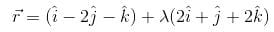is parallel to the plane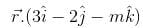, then the value of m is

JEE Advanced Level Test: Vector Algebra- 2 - Question 2

### Given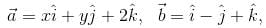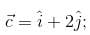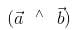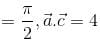, then

JEE Advanced Level Test: Vector Algebra- 2 - Question 3

###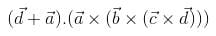simplifies to

JEE Advanced Level Test: Vector Algebra- 2 - Question 4

Let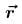be a vector perpendicular to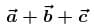, where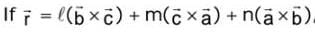then (ℓ +m+n) is equal to

JEE Advanced Level Test: Vector Algebra- 2 - Question 5

If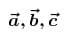are three non-coplaner non-zero vectors and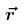is any vector in space, then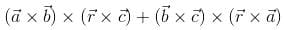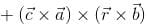is equal to

JEE Advanced Level Test: Vector Algebra- 2 - Question 6

If a, b, c are pth, qth, rth terms of an H.P and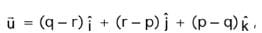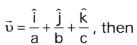JEE Advanced Level Test: Vector Algebra- 2 - Question 7

If the unit vectors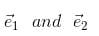are inclined at an angle 2θ and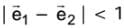, then for θ ∈ [0, π], θ may lie in the interval

JEE Advanced Level Test: Vector Algebra- 2 - Question 8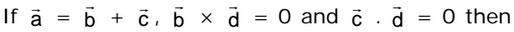JEE Advanced Level Test: Vector Algebra- 2 - Question 9

Consider a tetrahedron with faces f1, f2, f3, fLet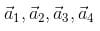​ be the vectors whose magnitudes are respectively equalt to the areas of  f1, f2, f3, f4 and  whose directions are perpendicular to these faces in the outward direction. Then

JEE Advanced Level Test: Vector Algebra- 2 - Question 10

Let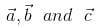be non-coplaner unit vectors equally inclined to one another at an acute angle θ. Then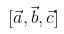in terms of  θ is equalt to

JEE Advanced Level Test: Vector Algebra- 2 - Question 11

Let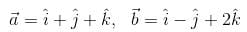and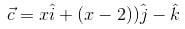.  If the vector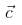lies in the plane of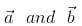, then x equals

JEE Advanced Level Test: Vector Algebra- 2 - Question 12

Images of the point P with position vector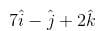in the line whose vector equation is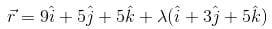has the position vector

JEE Advanced Level Test: Vector Algebra- 2 - Question 13

Let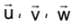be such that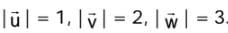. If the projection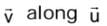is equal to that of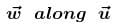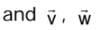are  perpendicular  to each other, then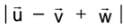equals.

JEE Advanced Level Test: Vector Algebra- 2 - Question 14

Let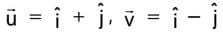and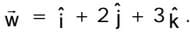. If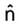is a unit vector such that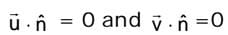then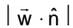is equal to

JEE Advanced Level Test: Vector Algebra- 2 - Question 15

Equation of a line which passes through a point with position vector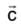, parallel to the plane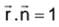and perpendicular to the line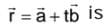JEE Advanced Level Test: Vector Algebra- 2 - Question 16

Points L, M and N lie on the sides AB, BC and CA of the triangle ABC such that ℓ(AL) : ℓ(LB) = ℓ(BM) :ℓ(MC) = ℓ(CN) : ℓ(NA) = m :n, then the areas of the triangles LMN and ABC are in the ratio

JEE Advanced Level Test: Vector Algebra- 2 - Question 17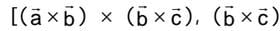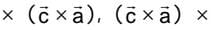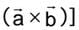is equal to

Detailed Solution for JEE Advanced Level Test: Vector Algebra- 2 - Question 17

Solution :- [a×b b×c c×a]

= (a×b) . [(b×c) * (c×a)]

= (a×b) [(b×c) . a(c) - (b×c) . c]

= (a×b) [(b×c) . a(c) - 0]

= [(a×b) . c] [(b×c) . a]

= [a b c] [(b c a]

= - [a b c] [(a c b]

= [a b c] [(a b c ]   => [a b c]^2

JEE Advanced Level Test: Vector Algebra- 2 - Question 18

If the vectors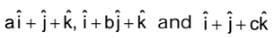(a ≠ b ≠ c ≠ 1) are coplanar, then the value of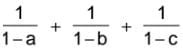is equal to

JEE Advanced Level Test: Vector Algebra- 2 - Question 19

The vectors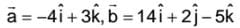are coinitial. The vector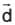which is bisecting the angle between the vectors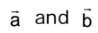and is having the magnitude √6 is

JEE Advanced Level Test: Vector Algebra- 2 - Question 20

If A1, A2, A3, .........Aare the vertics of a regular plane polygon with n sides and O is its centre then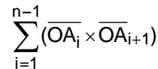equals

JEE Advanced Level Test: Vector Algebra- 2 - Question 21

For any four points P,Q, R, S,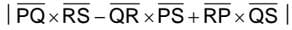is equal to 4 times the

area of the triangle

*Multiple options can be correct
JEE Advanced Level Test: Vector Algebra- 2 - Question 22

If a line has a vector equation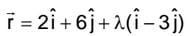, then which of the following statements hold goods ?

*Multiple options can be correct
JEE Advanced Level Test: Vector Algebra- 2 - Question 23

The vector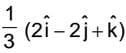is

*Multiple options can be correct
JEE Advanced Level Test: Vector Algebra- 2 - Question 24

The vector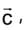directed along the external bisector of the angle between the vectors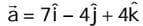and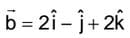with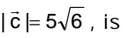JEE Advanced Level Test: Vector Algebra- 2 - Question 25

Which of the following acts in accordance with right hand screw rule?(Scan QR code)